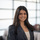# Using Python to Plot only the Rolling Average Line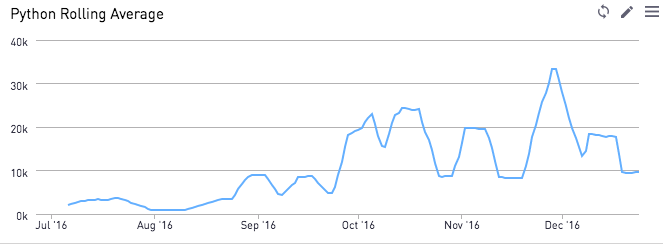Want to show a rolling average to your data? Using the Periscope Data Python / R integration, we can accomplish this with a single line of Python code.

Here are the first few rows of our SQL output - a list of dates with the number of users created on a fictional gaming platform.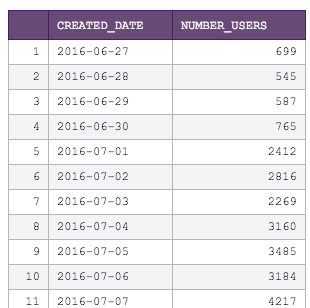We now use the rolling() function from Python pandas, as shown below.  Note that by changing the .mean() to a .sum(), we can now make this a rolling sum calculation. Neat, huh?

```# SQL output is imported as a pandas dataframe variable called "df"
import pandas as pd

df["rolling"] = df["number_users"].rolling(10).mean()

# Use Periscope to visualize a dataframe or an image by passing data to periscope.output()
periscope.output(df)```

Now, we make this chart a line graph to visualize the rolling average line by itself!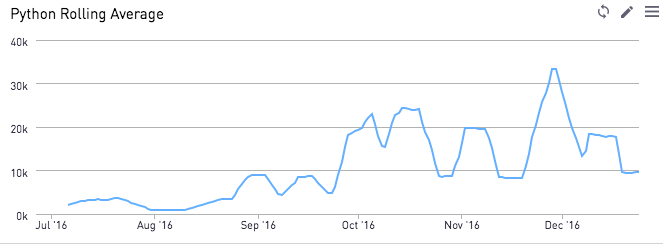Tip: If you want to display both the raw data and the rolling average, Periscope's built-in visualizations has a quick "Show Rolling Average" check box that you can toggle on.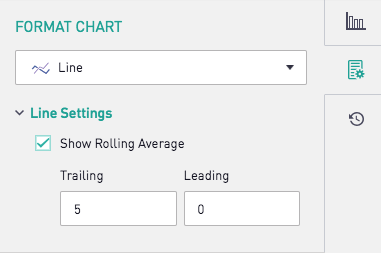Want to stick to SQL? You can run the same calculation using SQL window functions as shown here

Prefer R? Find the R equivalent for this post here